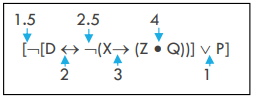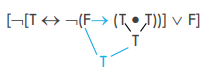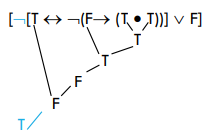# 6.4: Logical Operators as Truth Functions

•• Andrew Lavin
• Butte College
$$\newcommand{\vecs}{\overset { \rightharpoonup} {\mathbf{#1}} }$$ $$\newcommand{\vecd}{\overset{-\!-\!\rightharpoonup}{\vphantom{a}\smash {#1}}}$$$$\newcommand{\id}{\mathrm{id}}$$ $$\newcommand{\Span}{\mathrm{span}}$$ $$\newcommand{\kernel}{\mathrm{null}\,}$$ $$\newcommand{\range}{\mathrm{range}\,}$$ $$\newcommand{\RealPart}{\mathrm{Re}}$$ $$\newcommand{\ImaginaryPart}{\mathrm{Im}}$$ $$\newcommand{\Argument}{\mathrm{Arg}}$$ $$\newcommand{\norm}{\| #1 \|}$$ $$\newcommand{\inner}{\langle #1, #2 \rangle}$$ $$\newcommand{\Span}{\mathrm{span}}$$ $$\newcommand{\id}{\mathrm{id}}$$ $$\newcommand{\Span}{\mathrm{span}}$$ $$\newcommand{\kernel}{\mathrm{null}\,}$$ $$\newcommand{\range}{\mathrm{range}\,}$$ $$\newcommand{\RealPart}{\mathrm{Re}}$$ $$\newcommand{\ImaginaryPart}{\mathrm{Im}}$$ $$\newcommand{\Argument}{\mathrm{Arg}}$$ $$\newcommand{\norm}{\| #1 \|}$$ $$\newcommand{\inner}{\langle #1, #2 \rangle}$$ $$\newcommand{\Span}{\mathrm{span}}$$$$\newcommand{\AA}{\unicode[.8,0]{x212B}}$$

## What’s a Truth Function?

As we delve a bit deeper in learning about our five logical operators, the next step is to explore exactly what they mean and how they work. We might have an intuitive sense of what they mean, but here we’ll solidify that understanding in a logically precise way.

If I tell you Barack Obama is president, then I’ve told you that the world is one determinate way. Alternatively, if I tell you that

Either Barack Obama is president Or he’s retired

then I’ve told you that we live in one of two possible worlds: one world where Obama is president right now or another world where Obama is retired right now. Which world do we actually live in?

Notice how this disjunctive statement is true or false depending on the truth or falsity of its parts. A fancy logician’s way of saying essentially the same thing is that the truth of the whole statement is a function of the truth of its parts. This statement is very clearly dependent on its parts:

I will both graduate high school and get into college

The statement as a whole is false if the person never graduates high school (even if they get into college!). It’s also a false statement is the person never gets into college (even if they graduate high school!). The statement as a whole is only going to be true if both of the conjuncts end up being true.

This is what it means to say that our logical operators ($$\neg$$, $$\wedge$$, $$\vee$$, $$\rightarrow$$, and $$\leftrightarrow$$) are Truth Functions or Truth Functional Operators—they are little machines that tell us whether a whole statement is true based on whether the component parts are true.

If I tell you that I’m either going to get rich or I’m going to get arrested, my statement will be true if I get rich, it will be true if I get arrested, and it will probably also count as true if I get rich and get arrested! It’s a disjunction, and disjunctions are true if just one of their disjuncts are true.

If I tell you that “I am not getting into UCLA,” but then I do get into UCLA, I have said something false, right? A negation is true if the negated statement is false, and false if the negated statement is true. If I say “I am not going to that party tonight,” and then I end up not showing up, then I have said something true: the proposition “I am going to that part tonight” ended up being false and so the negated version of it ends up being true.

If I tell you that “you’ll get your allowance if and only if you do your chores,” but then you do your chores and don’t get your allowance, then my statement will have been false: turns out doing your chores wasn’t perfectly sufficient for getting your allowance.

Each operator, in other words, takes certain inputs (the truth or falsity of the atomic sentences that make up its disjuncts, conjuncts, antecedent and consequent, or the like) and delivers one output: a true or a false. This is a logical operation. It’s sort of like each of our five logical operators are little machines.

Think about it like a vending machine:I need to input the correct set of inputs to get the output I want. This is because every set of inputs corresponds to a particular output. If I put money in and then push “D-7”, I’ll get a soda, but if I put a different amount of money in and then push “G-8”, I’ll get a candy bar. Different inputs means a different output.

Now for Logical Operators, we only have two possible outputs: True and False. These two values are called “truth values”. It’s a bit confusing because only one of them is “true”, but that’s what they’re called. If it’s helpful, think of them like numerical values. If I am writing a number in the standard decimal system, then I can fill in values 0-9 for the 1’s place, the 10’s place, the 100’s place, and so on. 0-9 are the values that can go into those places. For instance, “106” has a ‘1’ in the hundreds place, a ‘0’ in the tens, and a ‘6’ in the ones places. Instead of ten different values, though, logic only works with two values: ‘true’ and ‘false.’ It’s kind of like binary, where ‘0’ and ‘1’ are the only values you have to work with.

If each operator gives an output of “true” or “false”, what sorts of inputs do we give into the system? Well, the inputs are more truth values. A conjunction takes two T’s and turns them into one output of T. A disjunction takes an input of T and F and outputs T. A negation takes an input of T and outputs F. Each operator takes some set of truth values as an input (1 or 2 truth values) and then outputs just one truth value. Note: negation is the only operator that takes just one input. The other four take two inputs.

A function is something that takes an input and gives you an output. So logical operators are Truth Functions in the sense that they take truth values as inputs and give you truth values as an output.

Truth functions work together in a particular order to determine what individual output will result from a set of inputs. So just as:

$$\neg$$ A

Is a truth function or a truth functional formula, so is:

$$\neg$$(A $$\leftrightarrow$$ B)

And also

[$$\neg$$[D $$\leftrightarrow$$ $$\neg$$(X$$\rightarrow$$ (Z $$\bullet$$ Q))] $$\vee$$ P]

It ain’t pretty, but it is a truth functional formula in that given a set of inputs (one input for each sentence letter), it will give exactly one output. The parentheses and brackets determine the order in which order we apply the operations, but we’ll get into that later. For now, just keep in mind that a set of inputs will give a single output for any WFF in propositional logic:

$\begin{array} \ D: False \\ X: True \\ Z: True \\ Q: False \\ P: True \end{array} \Rightarrow [\neg [D \leftrightarrow \neg ( X \rightarrow (Z \bullet Q ))] \vee P ] \Rightarrow True \nonumber$

## What’s a Truth Table?

A truth table is just that: a table of truth values. It tells you what output you’ll see given some set of inputs. Let’s look at a very simple one so we can see what the different parts are:Figure $$\PageIndex{1}$$: Anatomy of a truth table

The left side of the truth table is called the “input side” because it houses the inputs that will determine what outputs we find on the right side or “output side”. A complete set of inputs and outputs is represented in each row of the truth table. The boxed row above has two T’s as inputs and a T as an output. Remember that there is always just one output in classical propositional logic, even though there might be lots of inputs.

The inputs are the truth values of the atomic sentence letters that make up part of the formula. The formula(s) that define(s) the truth table are found on the top right heading above the outputs.

So what this table is telling us is that if A and B are both Truth, then (A $$\vee$$ B) will be true. Similarly if A is True and B is False (second row) or if A is False and B is True (third row). But if (fourth row) A and B are both False, then (A $$\vee$$ B) will be false as well.

## Definitions of Logical Operators

The following table summarizes the “truth functional profile” of each operator: it tells you what the output will be given various inputs.

A B ~B (A$$\bullet$$B) (A$$\vee$$B) (A$$\rightarrow$$B) (A$$\leftrightarrow$$B)
T T F T T T T
T F T F T F F
F T   F T T F
F F   F F T T

How do we read this table? It’s easier than it looks. That thick vertical white line between “B” and “~B” divides the inputs from the outputs. So the “A” and “B” columns are the inputs columns and the rest are telling us what the output of various operators will be. The simplest column is “~B”: it tells us that when the proposition being negated is True (first row where B is “T”), ~B will be False, and when the proposition being negated is False (second row where B is “F”), ~B will be True.

The other columns are a bit trickier since they involve the inputs of a truth value for B and a truth value for A. The (A$$\bullet$$B) column is telling us that (A$$\bullet$$B) is true only when both inputs are “T”s. Otherwise, it’s false.

I’ve highlighted the “odd one out” for each column. This is the easiest way to memorize this information: figure out what the exception to the rule is and memorize that. (A$$\bullet$$B) is false for all but one set of inputs (T and T). (A$$\vee$$B) is true for all but one set of inputs (F and F). (A$$\rightarrow$$B) is true for all but one set of inputs (T and F, in that order). Finally, (A$$\leftrightarrow$$B) is true when the inputs are the same and false otherwise.

Here’s a different way of representing the same information:

• ~A is the opposite of A
• (A$$\bullet$$B) is true only when both are true
• (AvB) is false only when both are false
• (A$$\supset$$B) is false only when A is T and B is F
• (A$$\equiv$$B) is true only when A and B are the same

## Computing Truth Values

Now that we know what each truth functional operator does, we can perform an operation: we can finally do something with logic.

How do we do it? It’s simple: we start from the inside and work our way out.

Here are the steps:

### Step One: Find the Innermost Operator

The parentheses and brackets enclose the operators. The more parentheses or brackets there are enclosing an operator, the further “inside” the formula the operator is. Our first task is to find the operator that is the most “inside” the formula. Let’s practice on this formula:

[$$\neg$$[D $$\leftrightarrow$$ $$\neg$$(X$$\rightarrow$$ (Z $$\bullet$$ Q))] $$\vee$$ P]

A fast way of doing this would be to count up how many sets of parentheses or brackets enclose each operator. For negations, we’ll pretend that there’s a 1/2 set of parentheses around them so we can be sure to get the order correct. I’ve counted them up here:Negations don’t technically have parentheses or brackets around them, but they also must be calculated before the operators that have the same amount of parentheses as they do. For instance, the $$\vee$$ and the leftmost $$\neg$$ are both inside of only one set of parentheses/brackets, but we are supposed to calculate the value of the negation before we calculate the disjunction. So we add an extra .5 as if there was an extra set of parentheses around the negation and what it negates.

What’s the innermost operator? It looks like that $$\bullet$$ is the innermost: it is enclosed by 4 sets of parentheses/brackets. The $$\rightarrow$$ is next, then the $$\neg$$ to the left of it. Then the $$\leftrightarrow$$, the leftmost $$\neg$$, and finally the Main Operator: the $$\vee$$.

The Main Operator is the operator that is enclosed by the outermost parentheses/brackets (or, if it’s a negation, it’s not enclosed at all). The truth value output of the main operator is the truth value of the whole proposition.

### Step Two: Fill in the given truth values

We are given the truth values for each atomic sentence. For instance “Barack Obama is President of Canada” is a false proposition, while “Barack Obama was President of the United States” is a true proposition. When we’re calculating these truth values, though, we are doing something that is “pure logic” in that it’s divorced from the actual truth or falsity of the propositions. At this point, let’s just pretend that these truth values come from a random flip of a coin. We’re trying to calculate what the truth value of the whole proposition will be given a random set of atomic truth values.

If you’re given a practice problem for this section, it will have truth values for each sentence letter given as part of the problem. Here is an example:

[$$\neg$$[D $$\leftrightarrow$$ $$\neg$$(X$$\rightarrow$$ (Z $$\bullet$$ Q))] $$\vee$$ P]

D, Z, and Q are True

X and P are False

We then, for step two, fill in these truth values like so:

[$$\neg$$[T $$\leftrightarrow$$ $$\neg$$(F$$\rightarrow$$ (T $$\bullet$$ T))] $$\vee$$ F]

Now we’re ready to start calculating!

### Step Three: Calculate from the “Inside-Out”

Now that we’ve identified the order of the operators, we can calculate the truth values from the inside out.

Each step here is as simple as looking up in the truth table from Definitions of Logical Operators the inputs you’re given and then writing down the output from the table. If you understanding the operators a bit more clearly, then you can skip the truth table and go straight to understanding what the output should be. We’ll do the painstaking version here just this once.

We decided in step one that the order is: $$\bullet$$, $$\rightarrow$$, the inner $$\neg$$, $$\leftrightarrow$$, the leftmost $$\neg$$, and finally $$\vee$$.

What surrounds the $$\bullet$$?

(T $$\bullet$$ T)

What is the truth table for $$\bullet$$?

A

B

(A$$\bullet$$B)

T

T

T

T

F

F

F

T

F

F

F

F

I’ve highlighted the relevant row already. The two inputs are T and T, so we want to look at the top row. What’s the output? T! We can now move forward in our calculation:Now to calculate the $$\rightarrow$$. What’s the input here? We take the F from before the arrow and the T that we calculated in our previous step. So it’s:

F$$\rightarrow$$T

Again, we consult the truth table:

A

B

(A$$\rightarrow$$B)

T

T

T

T

F

F

F

T

T

F

F

T

Looks like the row we’re interested in is the 3rd row: F$$\rightarrow$$T. What’s the output? T! That T is the truth value of everything inside the parentheses there, so it’s the truth value of (F$$\rightarrow$$ (T $$\bullet$$ T)). Again, we can mark this on our formula:What operator is next? The negation just to the left of that arrow. In this case the negation negates everything in those parentheses to its right (F$$\rightarrow$$ (T $$\bullet$$ T)), which means it negates that T we just calculated.

What’s the truth table for Negation?

A ~A
T F
F T

If we’re calculating $$\bf\neg$$T, then we check to see what the output will be when the input is T. So in the table above, when A is T, ~A is F. That means $$\neg$$(F$$\rightarrow$$ (T $$\bullet$$ T)) is False! Again, we update our formula:We can now calculate the truth value of the $$\leftrightarrow$$. What are it’s inputs? It has T to the left and to the right? ... an F. Good job. What is T$$\leftrightarrow$$F? Look it up in the truth table:

A

B

(A$$\leftrightarrow$$B)

T

T

T

T

F

F

F

T

F

F

F

T

A “$$\leftrightarrow$$” is true only when both sides are the same. Are both sides the same in T$$\leftrightarrow$$F? No, of course not. It follows that T$$\leftrightarrow$$F is False. Again, update our formula:Almost done! Calculate the truth value of the negation. Remember: negation just flips whatever it negated. A T to an F and an F to a T. So what would $$\neg$$F be? Yep! True.Last step!!!!! The $$\vee$$ connects disjunct $$\neg$$[T $$\leftrightarrow$$ $$\neg$$(F$$\rightarrow$$ (T $$\bullet$$ T))] with disjunct F. We now know that the truth value of $$\neg$$[T $$\leftrightarrow$$ $$\neg$$(F$$\rightarrow$$ (T $$\bullet$$ T))] is True. What is T $$\vee$$ F? Pull up the Truth Table:

A

B

(A$$\vee$$B)

T

T

T

T

F

T

F

T

T

F

F

F

T $$\vee$$ F is the second row here: the output is True. Waaaay back in step one we decided that the main operator was the $$\vee$$, so that means we’re done once we’ve calculated the truth value of the $$\vee$$. We just did! It’s True.

What did we find out? We found out that [$$\neg$$[D $$\leftrightarrow$$ $$\neg$$(X$$\rightarrow$$ (Z $$\bullet$$ Q))] $$\vee$$ P] is True in the case that Propositions D, Z, and Q are True and Propositions X and P are False. The more we understand the definitions of the logical operators discussed in 6.4.3, the faster we’ll become at calculating complex truth values. Pretty soon it will become second nature.

This page titled 6.4: Logical Operators as Truth Functions is shared under a CC BY 4.0 license and was authored, remixed, and/or curated by Andrew Lavin via source content that was edited to the style and standards of the LibreTexts platform; a detailed edit history is available upon request.